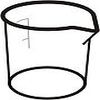#### You may also like### Brimful

Can you find the volumes of the mathematical vessels?

# Brimful 2

##### Age 16 to 18Challenge Level

The $y$-axis is an asymptote for the following curves:
$$y =- \frac{1}{x} \quad\quad y= -\frac{1}{x^2} \quad\quad y^2= \frac{1}{x^3}\quad\quad y = \ln(x)-1$$
Imagine rotating the $x> 0, y< -1$ regions of these curves about the $y$-axis to form a set of hollow vessels. Which vessels are of finite volume?

Numerical extension questions

1. Imagine that someone wishes accurately to engineer flasks for which the interior surfaces are given by these equations. They are to be truncated with a sealed base of radius $1$ micron, and a top opening of radius $1$cm. What would be their storage capacites and sizes? Are the resulting sizes such that you could envisage good approximations to such flasks being practically possible to make?
2. Imagine that similar vessels are made with truncated and open tops (of radius $1$cm) and bottoms.They are to be designed so that they do not leak when filled with water. How long would such flasks need to be? (Assume that the diameter of a water molecule is $3$nm)
3. Imagine that such flasks are made with open narrow ends of diameter $4$nm. Water is forcibly pumped into the flasks at a rate of $1$cm$^3$ s$^{-1}$, to create a jet of water consisting of a single water molecule. How fast would such jets emerge?